Question

# A student was required to prepare 250.0 mL of a hypochlorous acid/sodium hypochlorite buffer in which...

A student was required to prepare 250.0 mL of a hypochlorous acid/sodium hypochlorite buffer in which the concentration of the weak acid component was 0.084 M and the concentration of the conjugate base was 0.02 M. The student was supplied with 0.441 M hypochlorous acid and 1.0M NaOH to perform this task. What volume (in mL) of the acid would the student need to prepare this buffer solution? Hint: assume that all of the conjugate base comes directly from the reaction of NaOH with the weak acid (in other words, there is negligible dissociation of the weak acid).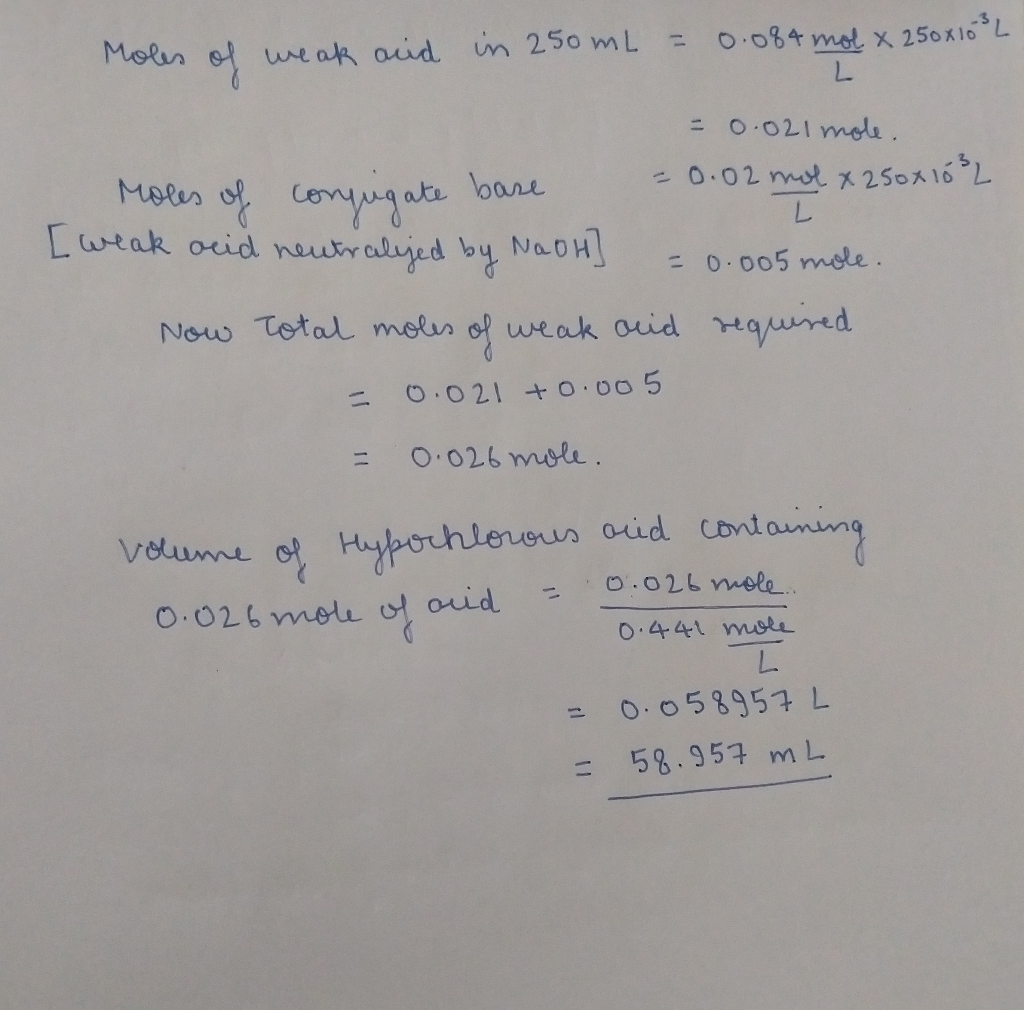#### Earn Coins

Coins can be redeemed for fabulous gifts.

Similar Homework Help Questions
• ### 1. What is the pH of a hypoiodous acid/sodium hypoiodite buffer in which the concentration of...

1. What is the pH of a hypoiodous acid/sodium hypoiodite buffer in which the concentration of the weak acid component is 0.129 M and the concentration of the conjugate base is 0.102 M?Ka=2.3E-11. 2. The pH of a hypoiodous acid/sodium hypoiodite buffer in which the concentration of the weak acid component is 0.140 M and the concentration of the conjugate base is 0.070 M is 10.34. Suppose 0.128 g of solid NaOH are added to 100 mL of this buffer...

• ### The weak acid of the buffer system is used to prepare the buffer by reacting with...

The weak acid of the buffer system is used to prepare the buffer by reacting with a strong base. The conjugated base is going to be obtained from some of the weak acid in reaction with NaOH 1M. This means you need to calculate the total amount of weak acid needed and the amount of 1.00 M NaOH solution you will have to add to create its conjugate form in solution.   Use 1.0M of each of these acids and basic...

• ### 2. A Student wants to prepare 250.0 mL of pH 4.1 buffer solution. He is planning...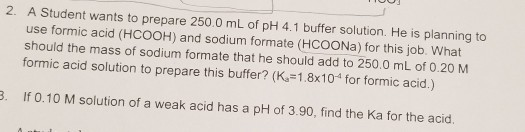2. A Student wants to prepare 250.0 mL of pH 4.1 buffer solution. He is planning to use formic acid (HCOOH) and sodium formate (HCOONa) for this job. What should the mass of sodium formate that he should add to 250,0 mL of 0.20 M formic acid solution to prepare this buffer? (K =1.8x10 for formic acid.) 3. If 0.10 M solution of a weak acid has a pH of 3.90, find the Ka for the acid.

• ### A buffer solution contains 0.355 M hypochlorous acid and 0.347 M sodium hypochlorite. If 0.0243 moles...

A buffer solution contains 0.355 M hypochlorous acid and 0.347 M sodium hypochlorite. If 0.0243 moles of hydrochloric acid are added to 125 mL of this buffer, what is the pH of the resulting solution ? (Assume that the volume does not change upon adding hydrochloric acid) pH=?

• ### A student must make a buffer solution with a pH of 2.00. Determine which weak acid...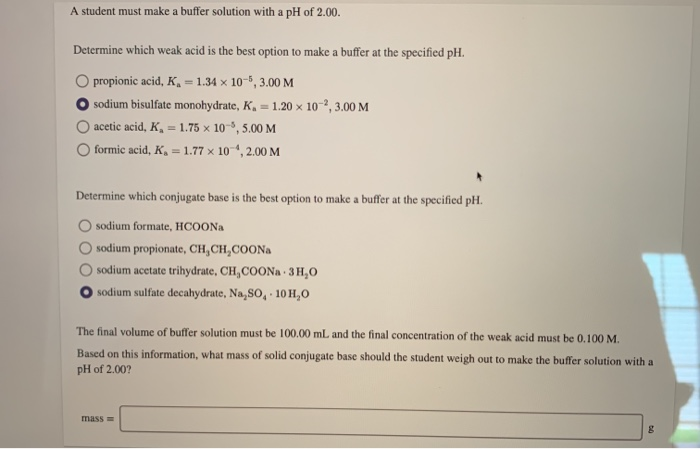A student must make a buffer solution with a pH of 2.00. Determine which weak acid is the best option to make a buffer at the specified pH. O propionic acid, K = 1.34 x 10-6, 3.00 M O sodium bisulfate monohydrate, K = 1.20 x 10,3.00 M acetic acid, K, = 1.75 x 10-6, 5.00 M formic acid, K, = 1.77 x 10 , 2.00 M Determine which conjugate base is the best option to make a buffer at...

• ### A student must make a buffer solution with a pH of 1.00. Determine which weak acid...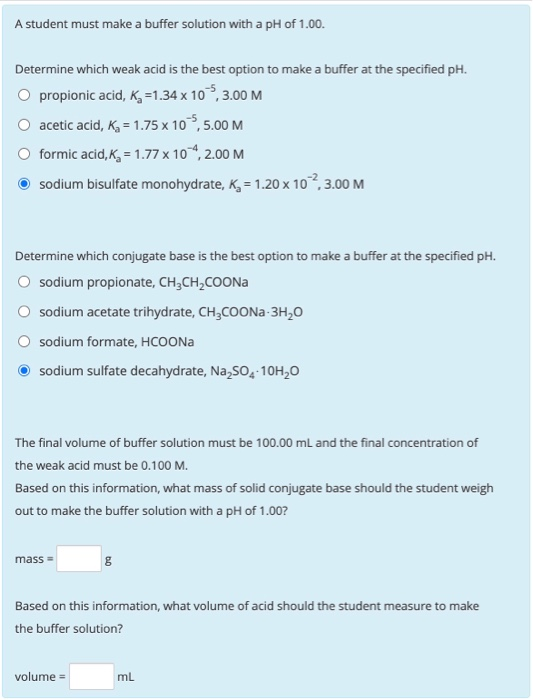A student must make a buffer solution with a pH of 1.00. Determine which weak acid is the best option to make a buffer at the specified pH. O propionic acid, K =1.34 x 10 5,3.00 M acetic acid, Ka = 1.75 x 10,5.00 M O formic acid, Kg = 1.77 x 104,2.00 M sodium bisulfate monohydrate, K = 1.20 x 102,3.00 M Determine which conjugate base is the best option to make a buffer at the specified pH. sodium...

• ### A student must make a buffer solution with a pH of 1.00, Determine which weak acid...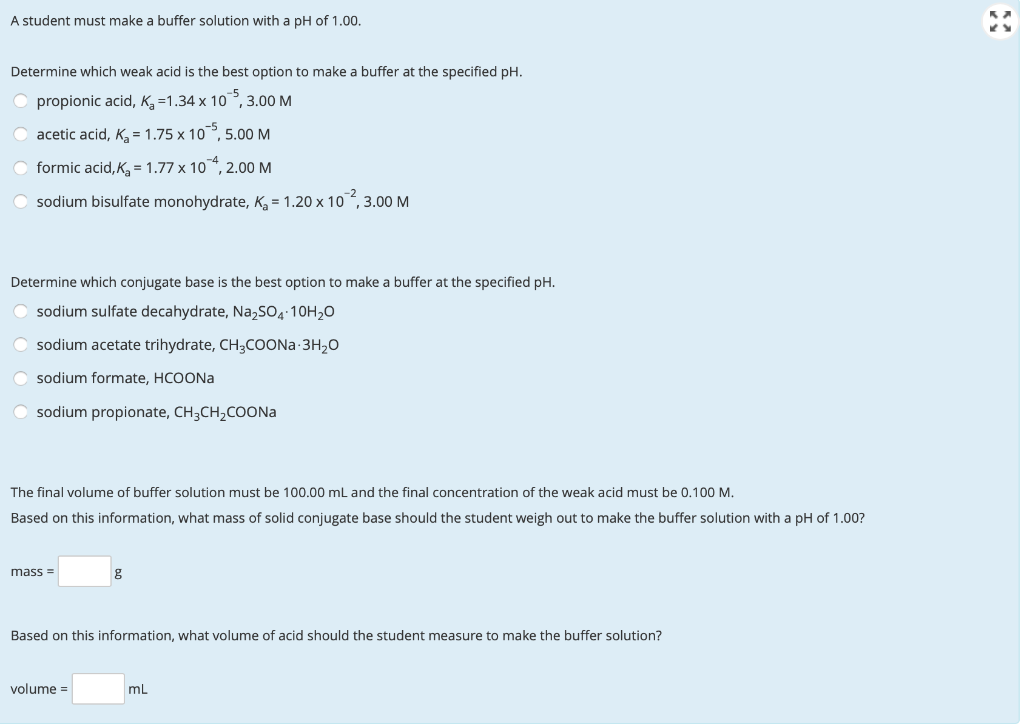A student must make a buffer solution with a pH of 1.00, Determine which weak acid is the best option to make a buffer at the specified pH. propionic acid, Kg =1.34 x 10,3.00 M acetic acid, Kg = 1.75 x 105,5.00 M formic acid, Kg = 1.77 x 104, 2.00 M sodium bisulfate monohydrate, K = 1.20 x 10,3.00 M Determine which conjugate base is the best option to make a buffer at the specified pH. sodium sulfate decahydrate,...

• ### Part 1: Buffers Considering the acetic acid sodium acetate buffer, complete the table below NaOH added/mL....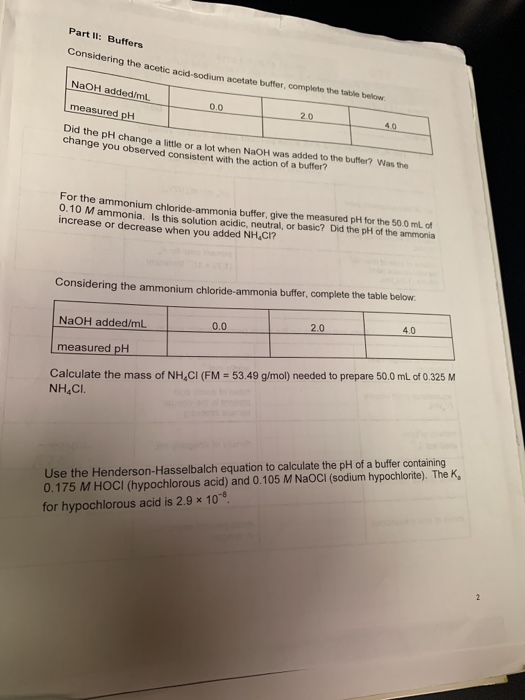Part 1: Buffers Considering the acetic acid sodium acetate buffer, complete the table below NaOH added/mL. measured pH 0.0 20 Did the pH change a little or a lot when NaOH was added to the butter? Was the change you observed consistent with the action of a butter? For the ammonium chloride-ammonia buffer give the measured pH for the 50.0 mL of 0.10 Mammonia. Is this solution acidic, neutral, or basic? Did the pH of the ammonia increase or decrease...

• ### How would you prepare 50.0 mL of a NaChi0Na CHO. buffer with pH-635 (give spec each...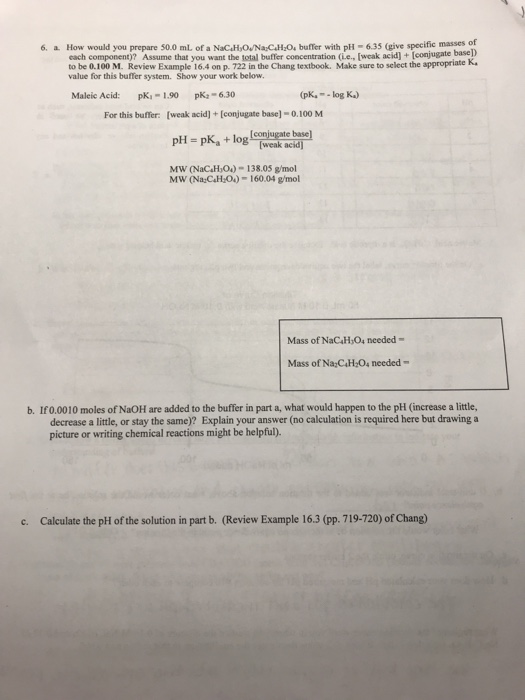How would you prepare 50.0 mL of a NaChi0Na CHO. buffer with pH-635 (give spec each component)? Assume that you want the total buffer concentration (Le, [weak acid]+ [conjugate ific masses of base]) 6、 a. to be 0.100 M. Review Example 16.4 on p. 72 in the Chang textbook. Make sure to select the sppropriate K- value for this buffer system. Show your work below. Maleic Acid: p-1.90 p-6.30 pk.--log K) [weak acid]+[conjugate base]- 0.100 M pH- pKa + MW...

• ### The pKa of hypochlorous acid is 7.530. A 56.0 mL solution of 0.112 M sodium hypochlorite...

The pKa of hypochlorous acid is 7.530. A 56.0 mL solution of 0.112 M sodium hypochlorite (NaOCl) is titrated with 0.252 M HCl. Calculate the pH of the solution: a) after the addition of 9.16 mL of 0.252 M HCl. . b) after the addition of 26.0 mL of 0.252 M HCl. c) at the equivalence point with 0.252 M HCl.